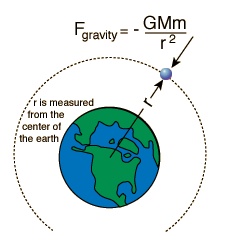# Gravitational Freefall

The freefall time and impact velocity for an object dropped from near the Earth can be calculated from simple trajectory equations if air friction can be neglected. But if the height is great enough for the acceleration of gravity to be significantly changed, then the calculation involves calculus to take into account the non-constant acceleration.This example is currently under development.

 Hyperbolic functions
Index

 HyperPhysics***** Mechanics R Nave
Go Back